# Excel logical operators: equal to, not equal to, greater than, less than

Many tasks you perform in Excel involve comparing data in different cells. For this, Microsoft Excel provides six logical operators, which are also called comparison operators. This tutorial aims to help you understand the insight of Excel logical operators and write the most efficient formulas for your data analysis.

## Excel logical operators - overview

A logical operator is used in Excel to compare two values. Logical operators are sometimes called Boolean operators because the result of the comparison in any given case can only be either TRUE or FALSE.

Six logical operators are available in Excel. The following table explains what each of them does and illustrates the theory with formula examples.

 Condition Operator Formula Example Description Equal to = =A1=B1 The formula returns TRUE if a value in cell A1 is equal to the values in cell B1; FALSE otherwise. Not equal to <> =A1<>B1 The formula returns TRUE if a value in cell A1 is not equal to the value in cell B1; FALSE otherwise. Greater than > =A1>B1 The formula returns TRUE if a value in cell A1 is greater than a value in cell B1; otherwise it returns FALSE. Less than < =A1= =A1>=B1 The formula returns TRUE if a value in cell A1 is greater than or equal to the values in cell B1; FALSE otherwise. Less than or equal to <= =A1<=B1 The formula returns TRUE if a value in cell A1 is less than or equal to the values in cell B1; FALSE otherwise.

The screenshot below demonstrates the results returned by Equal to, Not equal to, Greater than and Less than logical operators: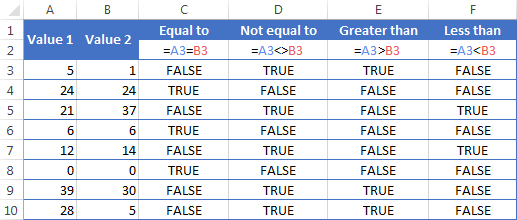It may seem that the above table covers it all and there's nothing more to talk about. But in fact, each logical operator has its own specificities and knowing them can help you harness the real power of Excel formulas.

### Using "Equal to" logical operator in Excel

The Equal to logical operator (=) can be used to compare all data types - numbers, dates, text values, Booleans, as well as the results returned by other Excel formulas. For example:

 =A1=B1 Returns TRUE if the values in cells A1 and B1 are the same, FALSE otherwise. =A1="oranges" Returns TRUE if cells A1 contain the word "oranges", FALSE otherwise. =A1=TRUE Returns TRUE if cells A1 contain the Boolean value TRUE, otherwise it returns FALSE. =A1=(B1/2) Returns TRUE if a number in cell A1 is equal to the quotient of the division of B1 by 2, FALSE otherwise.

#### Example 1. Using the "Equal to" operator with dates

You might be surprised to know that the Equal to logical operator cannot compare dates as easily as numbers. For example, if the cells A1 and A2 contain the date "12/1/2014", the formula `=A1=A2` will return TRUE exactly as it should.

However, if you try either `=A1=12/1/2014` or `=A1="12/1/2014"` you will get FALSE as the result. A bit unexpected, eh?

The point is that Excel stores dates as numbers beginning with 1-Jan-1900, which is stored as 1. The date 12/1/2014 is stored as 41974. In the above formulas, Microsoft Excel interprets "12/1/2014" as a usual text string, and since "12/1/2014" is not equal to 41974, it returns FALSE.

To get the correct result, you must always wrap a date in the DATEVALUE function, like this `=A1=DATEVALUE("12/1/2014")`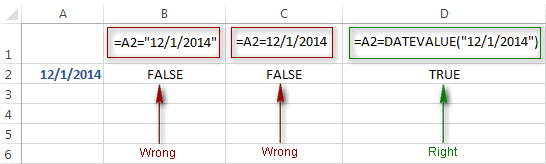Note. The DATEVALUE function needs to be used with other logical operator as well, as demonstrated in the examples that follow.

The same approach should be applied when you use Excel's equal to operator in the logical test of the IF function. You can find more info as well as a few formula examples in this tutorial: Using Excel IF function with dates.

#### Example 2. Using the "Equal to" operator with text values

Using Excel's Equal to operator with text values does not require any extra twists. The only thing you should keep in mind is that the Equal to logical operator in Excel is case-insensitive, meaning that case differences are ignored when comparing text values.

For example, if cell A1 contains the word "oranges" and cell B1 contains "Oranges", the formula `=A1=B1` will return TRUE.

If you want to compare text values taking in to account their case differences, you should use the EXACT function instead of the Equal to operator. The syntax of the EXACT function is as simple as:

EXACT(text1, text2)

Where text 1 and text2 are the values you want to compare. If the values are exactly the same, including case, Excel returns TRUE; otherwise, it returns FALSE. You can also use the EXACT function in IF formulas when you need a case-sensitive comparison of text values, as shown in the below screenshot: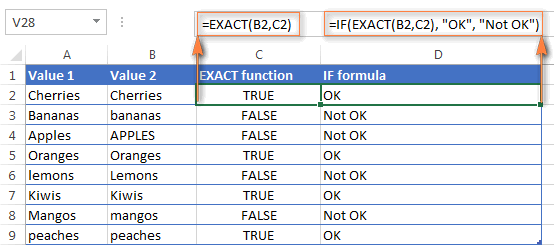Note. If you want to compare the length of two text values, you can use the LEN function instead, for example `=LEN(A2)=LEN(B2)` or `=LEN(A2)>=LEN(B2)`.

#### Example 3. Comparing Boolean values and numbers

There is a widespread opinion that in Microsoft Excel the Boolean value of TRUE always equates to 1 and FALSE to 0. However, this is only partially true, and the key word here is "always" or more precisely "not always" : )

When writing an 'equal to' logical expression that compares a Boolean value and a number, you need to specifically point out for Excel that a non-numeric Boolean value should be treated as a number. You can do this by adding the double minus sign in front of a Boolean value or a cell reference, e. g. `=A2=--TRUE` or `=A2=--B2`.

The 1st minus sign, which is technically called the unary operator, coerces TRUE/FALSE to -1/0, respectively, and the second unary negates the values turning them into +1 and 0. This will probably be easier to understand looking at the following screenshot: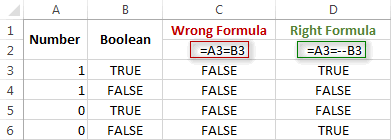Note. You should add the double unary operator before a Boolean when using other logical operators such as not equal to, greater than or less than to correctly compare a numeric and Boolean values.

When using logical operators in complex formulas, you might also need to add the double unary before each logical expression that returns TRUE or FALSE as the result. Here's an example of such a formula: SUMPRODUCT and SUMIFS in Excel.

### Using "Not equal to" logical operator in Excel

You use Excel's Not equal to operator (<>) when you want to make sure that a cell's value is not equal to a specified value. The use of the Not equal to operator is very similar to the use of Equal to that we discussed a moment ago.

The results returned by the Not equal to operator are analogous to the results produced by the Excel NOT function that reverses the value of its argument. The following table provides a few formula examples.

 Not equal to operator NOT function Description =A1<>B1 =NOT(A1=B1) Returns TRUE if the values in cells A1 and B1 are not the same, FALSE otherwise. =A1<>"oranges" =NOT(A1="oranges") Returns TRUE if cell A1 contains any value other than "oranges", FALSE if it contains "oranges" or "ORANGES" or "Oranges", etc. =A1<>TRUE =NOT(A1=TRUE) Returns TRUE if cell A1 contains any value other than TRUE, FALSE otherwise. =A1<>(B1/2) =NOT(A1=B1/2) Returns TRUE if a number in cell A1 is not equal to the quotient of the division of B1 by 2, FALSE otherwise. =A1<>DATEVALUE("12/1/2014") =NOT(A1=DATEVALUE("12/1/2014")) Returns TRUE if A1 contains any value other than the date of 1-Dec-2014, regardless of the date format, FALSE otherwise.

### Greater than, Less than, Greater than or equal to, Less than or equal to

You use these logical operators in Excel to check how one number compares to another. Microsoft Excel provides 4 comparison operates whose names are self-explanatory:

• Greater than (>)
• Greater than or equal to (>=)
• Less than (<)
• Less than or equal to (<=)

Most often, Excel comparison operators are used with numbers, date and time values. For example:

 =A1>20 Returns TRUE if a number in cell A1 is greater than 20, FALSE otherwise. =A1>=(B1/2) Returns TRUE if a number in cell A1 is greater than or equal to the quotient of the division of B1 by 2, FALSE otherwise. =A1

#### Using Excel comparison operators with text values

In theory, you can also use the greater than, greater than or equal to operators as well as their less than counterparts with text values. For example, if cell A1 contains "apples" and B1 contains "bananas", guess what the formula `=A1>B1` will return? Congratulations to those who've staked on FALSE : )

When comparing text values, Microsoft Excel ignores their case and compares the values symbol by symbol, "a" being considered the lowest text value and "z" - the highest text value.

So, when comparing the values of "apples" (A1) and "bananas" (B1), Excel starts with their first letters "a" and "b", respectively, and since "b" is greater than "a", the formula `=A1>B1` returns FALSE.

If the first letters are the same, then the 2nd letters are compared, if they happen to be identical too, then Excel gets to the 3rd, 4th letters and so on. For example, if A1 contained "apples" and B1 contained "agave", the formula `=A1>B1` would return TRUE because "p" is greater than "g".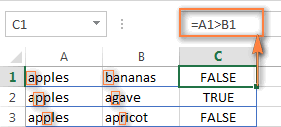At first sight, the use of comparison operators with text values seems to have very little practical sense, but you never know what you might need in the future, so probably this knowledge will prove helpful to someone.

## Common uses of logical operators in Excel

In real work, Excel logical operators are rarely used on their own. Agree, the Boolean values TRUE and FALSE they return, though very true (excuse the pun), are not very meaningful. To get more sensible results, you can use logical operators as part of Excel functions or conditional formatting rules, as demonstrated in the below examples.

#### 1. Using logical operators in arguments of Excel functions

When it comes to logical operators, Excel is very permissive and allows using them in parameters of many functions. One of the most common uses is found in Excel IF function where the comparison operators can help to construct a logical test, and the IF formula will return an appropriate result depending on whether the test evaluates to TRUE or FALSE. For example:

`=IF(A1>=B1, "OK", "Not OK")`

This simple IF formula returns OK if a value in cell A1 is greater than or equal to a value in cell B1, "Not OK" otherwise.

And here's another example:

`=IF(A1<>B1, SUM(A1:C1), "")`

The formula compares the values in cells A1 and B1, and if A1 is not equal to B1, the sum of values in cells A1:C1 is returned, an empty string otherwise.

Excel logical operators are also widely used in special IF functions such as SUMIF, COUNTIF, AVERAGEIF and their plural counterparts that return a result based on a certain condition or multiple conditions.

You can find a wealth of formula examples in the following tutorials:

#### 2. Using Excel logical operators in mathematical calculations

Of course, Excel functions are very powerful, but you don't always have to use them to achieve the desired result. For example, the results returned by the following two formulas are identical:

IF function: `=IF(B2>C2, B2*10, B2*5)`

Formula with logical operators: `=(B2>C2)*(B2*10)+(B2<=C2)*(B2*5)`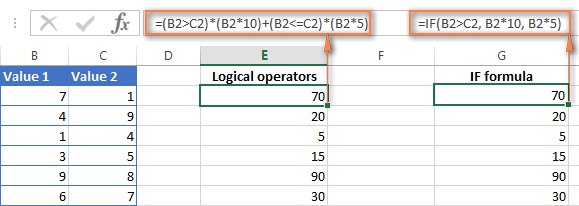I guess the IF formula is easier to interpret, right? It tells Excel to multiply a value in cell B2 by 10 if B2 is greater than C2, otherwise the value in B1 is multiplied by 5.

Now, let's analyze what the 2nd formula with the greater than and less than or equal to logical operators does. It helps to know that in mathematical calculations Excel does equate the Boolean value TRUE to 1, and FALSE to 0. Keeping this in mind, let's see what each of the logical expressions actually returns.

If a value in cell B2 is greater than a value in C2, then the expression B2>C2 is TRUE, and consequently equal to 1. On the other hand, B2<=C2 is FALSE and equal to 0. So, given that B2>C2, our formula undergoes the following transformation: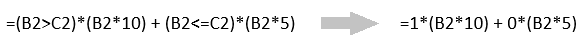Since any number multiplied by zero gives zero, we can cast away the second part of the formula after the plus sign. And because any number multiplied by 1 is that number, our complex formula turns into a simple =B2*10 that returns the product of multiplying B2 by 10, which is exactly what the above IF formula does : )

Obviously, if a value in cell B2 is less than in C2, then the expression B2>C2 evaluates to FALSE (0) and B2<=C2 to TRUE (1), meaning that the reverse of the described above will occur.

#### 3. Logical operators in Excel conditional formatting

Another common use of logical operators is found in Excel Conditional Formatting that lets you quickly highlight the most important information in a spreadsheet.

For example, the following simple rules highlight selected cells or entire rows in your worksheet depending on a value in column A:

Less than (orange): `=A1<5`

Greater than (green): `=A1>20`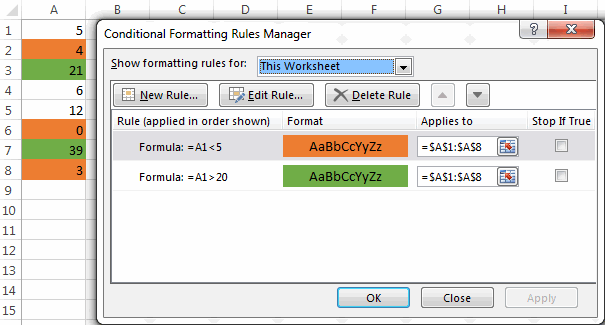For the detailed-step-by-step instructions and rule examples, please see the following articles:

As you see, the use of logical operators in Excel is intuitive and easy. In the next article, we are going to learn the nuts and bolts of Excel logical functions that allow performing more than one comparison in a formula. Please stay tuned and thank you for reading!

-->

### 999 Responses to "Excel logical operators: equal to, not equal to, greater than, less than"

1.HAPPY says:

21080931
21079882
21079117
21080035
21234
21225
22225
21228
I WANT TO ADD DOT (.)AFTER 2 NUMBERWHICH LEN IS GREATER THAN 6 NO
EX=
21.07.98
21.07.9117
21.08.0035
21.234
21.225
22.225
21.228

•AMIT says:

21080931 =IF((LEN(A1)<6),LEFT(A1,2)&"."&MID(A1,3,20),LEFT(A1,2)&"."&MID(A1,3,2)&"."&MID(A1,5,20))

2.Charlie says:

The formula you offered of course works on that cell, thank you! But I am wondering if I have a whole column of say 3000 entries in column A with say 50 different words that I am looking to label, the IF statement will get very long.

Excel suggests to use IFS statements instead of IF statements, but as you say, you can't use an IFS statement when searching for a substring.

Trying to find a practical solution...

3.Charlie says:

I did some research and found that this explains it very well using an array.
https://exceljet.net/formula/categorize-text-with-keywords

4.Malissa says:

I have an IF statement that looks at one cell and depending on what "class code" I have in that cell it refers to another cell then adds that number into the calculation. This is working fine but I want it to then put 0 instead of a negative number. I tried adding If(<0,0,) but it is not working and I have tried several variations of less than zero. Any suggestions?

•Doug says:

Malissa:
Can you post the formula that was working?

5.Dildar says:

Hi
I need kind help in this situation, i want excel formula:-

"Percentage apply on
Minimum " Maximum Tax amount Difference
Amount Amount Fixed Minimum and Maximum"
4,800,001 To 99,900,000,000 300,000 15.0%
2,400,001 To 4,800,000 60,000 10.0%
1,200,001 To 2,400,000 - 5.0%
800,001 To 1,200,000 2,000
400,001 To 800,000 1,000
Zero To 400,000 -

Note { Provided that where the taxable income exceeds Rs. 800,000/- the Minimum tax payable shall be Rs. 2,000/- }

6.AMIT ROCHLANI says:

IF(E8>60<90,F8,0)

THIS FORMULA NOT GIVEING MY ANSWER

•Mary Trifuntova (Ablebits.com Team) says:

Hello, Amit,

=IF((AND(E8>60, E8<90)), F8, 0)

Please also have a look at the following article on our blog:
Excel IF statement with multiple AND/OR conditions

Hope you'll find this information helpful.

•rahul says:

great

•JOHN says:

=IF((AND(E8>60, E84,R5<=4)),AE5,VLOOKUP(AE5,VALIDATION!\$M\$3:\$P\$24,4))

7.Sazzad says:

IF A1=25001 to 50000 & B1=yeas than C1=D1*10%, IF not match C1= 0
IF A1=50001 to 75000 & B1=yeas than C1=D1*15%, IF not match C1= 0

8.Manu says:

I applied a formula like
=A1
then it shows whatever is there in A1 . But if A1 is blank then its showing 0 instead of blank.
I want it blank if it is blank in A1

•Mary Trifuntova (Ablebits.com Team) says:

Hello, Manu.

=IF(ISBLANK(A1), "", A1)

Hope this is what you need.

9.Dan says:

Hi there,

trying to write an excel formula for the following:-

if a1 is greater than or less than b2 by more than 5% then colour cell? Any help would be greatly appreciated.

10.Stephanie Miller says:

I have a column that has taken the difference between two columns.

I want to extract any variances greater than 10K (so more than 10K or less than negative 10K) AND greater than 10% or less than negative 10%

I just want it go return that number if both conditions are met - or return a blank

What is the easy way to look at any difference more than a certain amount - whether that be negative or positive?

11.Abdul says:

Hi,
I have a set of number in a columns from 12 to 30 & I want result for them in 2 word
that can be more than 15 or less than 15. Is there a way I can get a formula result for these number. thank you

•Doug says:

Abdul:
If I understand your question an IF THEN OTHERWISE statement will work for you. Where the first number is in A2 enter this in B2:
=IF(A2<15,"Less Than 15","More Than 15")
It says, if the value in A2 is less than 15 then display "Less Than 15" otherwise display "More Than 15".

12.sterling says:

i need a formula for a completion time for each department for example IF any department goes 20 min ahead or behind produce which is F2 highlight Red if all departments are going to finish within 20 min of each other highlight Green my range is F2-F8

13.Wallace says:

If I got many data to lookup, how to enter the data by using if formula?

Ex: =if(A1>=1000,900,if(A1>=900,850........and so on)

14.OM BOPCHE says:

Que. If A1>B1 & C1=D1 then bonus will be (A1*0.002), but If A1>B1 & C1=D1 and F1>G1 & H1=I1 then bonus will be ((A1+F1)*0.002*2). Please suggest correct formula to be used?

•Mike says:

Hello, a formula that would work for you is the following:
=IF(AND(A1>B1, C1=D1, F1>G1, H1=I1), ((A1+F1)*0.002*2), IF(AND(A1>B1, C1=D1), A1*0.002, 0))

I used the information from https://www.ablebits.com/office-addins-blog/2018/06/06/excel-nested-if-statement/ to help come up with this answer.

15.Pauline Kearns says:

I need a formula that figures out how many entries in a range and then divides by that about of entries. Example if A1= 0, A2, = 3, A4=5 then divide by 2.

Thank you for your help. I actually have 7 entries - 1 for each day of the week, and want to divide only by the days that actually have a number greater than 0. So what I need is the sum of the 7 days then divide by only the number that had an actual value greater than 0.

Thank you!

•Mike says:

Hi Pauline, I hope you already received a response, but if not here's my answer. You would use the Countif function. It would look like this:
=COUNTIF(B1:B3,">0") the function looks at the cells B1 to B3 and returns a number between 0 and 3 depending on how many of the cells have a value higher than 0.

16.Tom Söräng says:

Hi Svetlana,

First I want to mention that I respect if you do not have the time to help and since you actually are making an income working with Excel I understand if you do not are able to help out.

I have searched on Internet "everywhere, but do not find the correct "programming" that works for me in my Excel sheet.

To start with, I work with time and a sheet using 24 hour and beyond this. Time can start at 21:00 and finish at 28:00 or 14:30 ending at 25:20 (that is the data I have (working hours and the extra amount of money we get working late)).

19-22 and 22-30
The rules I try to set is to find time that passes 19:00 and ends before 30:00 (06:00). Also I want to know if how much time is between 19:00 - 22:00 and how much is between 22:00 and 30:00.

Break (no extra payment)
For an example working hours are 14:00-23:30. Then we get three hours of extra payment between 19:00-22:00 and a higher amount of money between 22:00-23:30. But we do not get paid that extra money, below I have added a break between 18.30-19:30.

First I want to extract the 19:30 into one cell, 22:00 to the next, after that also 22:00 separately and at the end 23:30.

This is how I want it to look...

C2-14:00 Work starts (actual data)
C3-23:30 Work ends (actual data)
C4-18:30 Break starts (actual data, no payment from this time)
C5-19:30 Break ends (actual data, no payment to this time)
C6-19:30 Start time of lower payment (=IF... ) to get this result
C7- 22:00 End time of lower payment (=IF... ) to get this result
C8-22:00 Start time of higher payment (=IF... ) to get this result
C9-23:30 End time of higher payment (=IF... ) to get this result

Also to make sure the formulas work if the working hours are between 16:00-25:02 break at 21:20-22:40, 21:30-29:50 break at 25:00, 24:01-31:00 or 23:40-28:00 breaks embedded at any time...
I have tried this, but could not make it work.

Will this take to much time to write formulas that makes this work?

Best regards

Tom S

17.Tom S says:

Hi Svetlana,

First I want to mention that I respect if you do not have the time to help and since you actually are making an income working with Excel I understand if you do not are able to help out.

I have searched on Internet "everywhere", but do not find the correct "programming" that works for me in my Excel sheet.

To start with, I work with time and a sheet using 24 hour and beyond this. Time can start at 21:00 and finish at 28:00 or 14:30 ending at 25:20 (that is the data I have (working hours and the extra amount of money we get working late)).

19-22 and 22-30
The rules I try to set is to find time that passes 19:00 and ends before 30:00 (06:00). Also I want to know if how much time is between 19:00 - 22:00 and how much is between 22:00 and 30:00.

Break (no extra payment)
For an example working hours are 14:00-23:30. Then we get three hours of extra payment between 19:00-22:00 and a higher amount of money between 22:00-23:30. But we do not get paid that extra money, below I have added a break between 18.30-19:30.

First I want to extract the 19:30 into one cell, 22:00 to the next, after that also 22:00 separately and at the end 23:30.

This is how I want it to look...

C2-14:00 Work starts (actual data)
C3-23:30 Work ends (actual data)
C4-18:30 Break starts (actual data, no payment from this time)
C5-19:30 Break ends (actual data, no payment to this time)
C6-19:30 Start time of lower payment (=IF... ) to get this result
C7- 22:00 End time of lower payment (=IF... ) to get this result
C8-22:00 Start time of higher payment (=IF... ) to get this result
C9-23:30 End time of higher payment (=IF... ) to get this result

Also to make sure the formulas work if the working hours are between 16:00-25:02 break at 21:20-22:40, 21:30-29:50 break at 25:00, 24:01-31:00 or 23:40-28:00 breaks embedded at any time...
I have tried this, but could not make it work.

Will this take to much time to write formulas that makes this work?

Best regards

Tom S

18.Md. Faruquzzaman says:

I have a data four cell in excel now i want to summation both of data but if i input one cell less then 9 or equal to any character the output will be zero. How can i solve this issue. plzz do the ans for the question.

19.Diana says:

Hi,

I have two results in excel: 37.9% and 29.8%, how to type formula to say that if both of them are within 1% then report mean, if the difference is greater then the preferred result is taken as lower of the two values.

Thank you

20.Ray says:

Hi,

I am trying to convert positive \$ value to negative value if that positive value for an account number greater than 4 but less than 5. Can you please let me know what formula can I use to get that result?
Thanks

21.James Jacon says:

My case is for my item analysis in school.

E.g. 35 students in a class

The table says if:
75% above of the class size is M
<75% to 50% of the class size is NM
<50% below of the class size is LM

Can you help me to create a formula for this case. Thank you.

•Mary Trifuntova (Ablebits.com Team) says:

Hello, James,
=IF(A1 >= 75%, "M", IF(A1 >= 50%, "NM", "LM"))

Hope you’ll find this information helpful.

22.Adeniyi says:

Hello,

How do i go about this; I am using this function =IF(I7=1,"NPE",IF(I7=2<2.9,"BAE",IF(I7=3<3.9,"AE",IF(I7=4<4.9,"SE",IF(I7=5,"EE",))))) and i am getting '0' as the 'value if true'. Pls help.

23.Thelma says:

Hi
My current formula is =IF(C21,D21/C21,"") what I need is if cell C21>D21by .50 then my percentage should be 100

Ex.
9hr worked / 8.50hr booked then 100% Completion

24.JK Singh says:

Dear mam, I am a beginner for excel formula. My query is that i have many values in in column C in different rows. My condition is that to find out this value as minimum of 30 or equal to 30 but not less than 15 in column D. it's a value not true or false ie
If in a row a value is 9 in column c, then come to 15 in column d, if it is 22 in column c, then come to 22 in column d and if 45 in column c, then come to 30 in column d. how and what formula, i can use for it.
thanks with regards,
JK Singh

25.bipin says:

Hi!
Need Ur Help .
IF cell A1 is having date is not equal to B1 having date or cell A1 is balnk then it is to be considered as C1 cell date

26.bipin says:

Formulas IF Cell A1 IS NOT EQUAL TO Cell B2 or Cell A1 is blank then it is to be considered as Cell C1 data

27.GURUNATHAN S says:

how to round if Rs 7851.50/- is 'above' round to Rs.7860/- and round if Rs. 7850.40/- below round to Rs.7850/- in excel formula

28.Md. Shamiul Kaisar says:

Dear Concern,

Good Morning.

I need a help regarding the issue.

Amount TK
1 to 400 5
401 to 1500 10
1501 to 5000 15
5001 to above 25

Thanking you,

Kaisar

29.Charlie says:

I trying to create 'conditional formating' to highight the cell red if it's not empty and lower than 3. However I got error when I "use a formula to determine which cell to format" as below:
=\$J5"",\$J5<3

I must have missed some basic rules and get the error. Looking for your kind assitance to advise the correct method.

Thanks

30.RON says:

Hi
I'm trying to make a formula for if B3 is > 0= "Post Firm" , B3 is <0 = "PRE FIRM"
Basically if its less than zero PRE FIRM, greater than 0 POST FRIM will populate.

31.Harris Awan says:

Hi All,
I need to create a formula Like. If cell2 value is greater than 50% of cell1 value. Like cell1 value is 20 and if cell2 value is greater than 10 (which is greater than 50% of cell one value) then it says yes. Kindly assist me.
Thank you!

32.harendra kumar says:

0-4, 7-10, 11-15 these are days
SECURITY DEBIT BAL 0-4 7-10 11-15 16-20 21-25 26-30 31-60 >60 Payment
264000 152717 152717
519000 413382 2500 410882
634000 2402376 703575 757620 776181 80000 70000 15000 835245
433000 143055 143055

hi excel we unable to less the one payemnt (835245) in diffrent coloums, kindly help. which formula i have to use

33.shubham says:

=IF(I18500,I18-500*0.1+40))

Pls help

34.shubham says:

=IF(I18500,I18-500*0.1+40))

35.3 says:

trying to come up with a formula stating if cell 1 = cell 2 and cell 3 then cell 4 is the answer

36.David says:

How can I conditionally format a cell if it requires 2 conditions to be meet. Also, both of the cells have to meet a secondary condition.

example:
Cell A1 to be formatted: Cell D3 must be greater than C3, and Cell T3 must be grater than cell S3.

37.Steven Hartley says:

It has been 20 years since I last took a boolean class...
I have a simple basic combination of IF(AND( >= to problems for you....
I am trying to make a simple entry to say: if the number in these three locations are ALL greater than 5 - then count it as one.

=IF(AND(B439>5,C439>5,D439>5),1,0) -

But here is the catch: I need zero to be counted as a ten place holder. So I need it to count all numbers in these locations which equal 6, 7, 8, 9, & 0.

At the other end of the stick - I also need it to count everything greater than 0 and less than 6. So I need it to count all numbers in these locations which equal 1, 2, 3, 4, & 5. Ignoring the zero...

But for some reason the combinations of Greater than and equal to refuse to give me the answer I need.... Help....

Can't seem to ever find the right examples of multiple combinations of nested Boolean...

•Steven Hartley says:

I tried this - but it doesn't work:
=IF(AND(B435=0,B435>=6,C435=0,C435>=6,D435=0,D435>=6),1,0)

This does seem to work with the count 1 to 5:
=IF(AND(B435>=1,B435=1,C435=1,D435<=5),1,0)

Not sure what th difference is - but there must be one. The bottom one works and the top one does not...

•Svetlana Cheusheva (Ablebits.com Team) says:

Hi Steven,

The difference is that the logical test in the first formula can never be TRUE because a number cannot be equal to 0 and greater than 6 at the same time :)
You need a combination of OR and AND statements in this case:
=IF(AND(OR(B435=0,B435>=6), OR(C435=0,C435>=6), OR(D435=0,D435>=6)), 1, 0)

•STEVE HARTLEY says:

MANY MANY THANKS! I was trying all kinds of versions - even played with NOT commands nothing worked. Your solution is GOD send.

38.Steven Hartley says:

That is so weird - that bottom one is not the formula I wrote - it changed when I hit enter... It said: =IF(AND(B435>=1,B435=1,C435=1,D435<=5),1,0)

39.Steven Hartley says:

WOW! It did it again....

•Svetlana Cheusheva (Ablebits.com Team) says:

Sorry for this. Our blog engine occasionally mangles formulas with "greater than" and "less than" operators, and our web-master can do nothing about it. Aargh...

40.Jagannath says:

If B2 contains a particular text then it should update E2 as Alert(text), how to update this logic

41.Jahedul says:

I am very happy to find out solve my problem from your mathematical logical part.
Thank you

42.M_CDV says:

Hello,

I am trying to do a comparison between serial numbers. To simplify my case, I would like to compare two numbers and to know if 00001 is superior to 50000.
The formula is =IF(00001>50000,2018,2019)
The result that I obtain is wrong and always returns 2018. Is there a way to do compare these two numbers in excel?

Thanks for your help on this.

Kind Regards,

43.Peter McEwan says:

I want a formula for:
If A1 is equal to or less than £50,000, then A2 = £60.
If A1 is equal to or less than £100,000, then A2= £120.
And so on and so forth...
£150,000=£240
£200,000=£360
£300,000=£480
£500,000=600
£700,000=720
£1,000,000=£840
£2,000,000=£1,000
£3,000,000=£3,000
£5,000,000=£5,000
<£5,000,000=£7,500

44.Mark says:

Hi there,
I have 2000 rows of survey response data that is numerically coded : e.g. 1=Positive and 2=Negative. I want to enumerate the number of positive and negative responses. My thoughts are to use an IF equation that says IF the cell =1 assign it a one and if the cell =2 assign it a zero. Then I can enumerate the sum total number of positives and deduct from all responses to get the number of negatives at the bottom of the columns.

Could you advise what formula I could use to get my numeric responses recoded as 1 and 0 instead of 1 and 2.

Many thanks

45.Abdul Wahab says:

Hi,
can you please explain how can i type greater than and less then symbol in excel formula or i have to download this symbol.
Regards
Abdul Wahab

•Svetlana Cheusheva (Ablebits.com Team) says:

Hi Abdul,

The 'greater than' and 'less than' symbols (also called angle brackets) are found on all computer keyboards. On U.S. keyboards, greater than (>) is usually located on the same key as the period and less than (<) on the same key as the comma.

46.lovely says:

please anyone immediate help me. i have some trouble in one formula is that :-
=IF(A1*0.2%-LEFT(A1*0.2%,2)>0.5,ROUNDUP(A1*0.2%,0),ROUNDDOWN(A1*0.2%,0))
this formula is correct. but i want to add one more condition is that the result value not greater than 25. i want that (this formula + not greater than 25) how to combine both them.

47.John says:

the criteria is
0% = 15, Between 0% and 3% = 9, Greater than 3% = 0
I tried the formula:
=IF(Q3=0,15,IF(AND(Q3>0,Q33,0),0)))
But it doesn't read the last argument which those that have more than 3% should be 0. It still shows 9.

48.Ranit Mehta says:

I want to some data in other sheet as greater than 1100 to less than 1500
1000
1100
1200
1300
1400
1500
1600
1450
1375
1890
1225
2550

49.Tyson says:

I need help please. I have a formula currently set up to calculate total hours, =((J18+TIME(0,30,0))-(H18-TIME(0,30,0)))*24. Now I need to set it up so that if that results in less than 3hrs for the cell to display 3 but if it is greater than 3 hrs to display the answer

50.cristian micu says:

i have this excel formula involving sumif with 2 columns, the test column is comprised of one letter having 3 possible values in column, the sum column has numbers

the formula is this =SUM(K13:K2005)/(SUMIF(J13:J2005, "", H13:H2005))
j colums is the test column with the letters, h is the value colums added with the numbers

my question is what "" means in this instance

thank you

•Svetlana Cheusheva (Ablebits.com Team) says:

Hi Cristian,

"" means an empty string. In other words, your SUMIF function says: if a cell in column J is empty, then add up a number in column H in the same row.

51.dhruv says:

if greater a1 less than or equals to 2 then e2 is 0.05 if a1 is greater than 2 then e2 is 0.03

52.Maria says:

how do i write formula for...
if c21 is more than 40, multiply what is more than 40 by 15.00

•Zonbrix says:

So what happen if c21 is no greater that 40?. Well, below formula leave the c21 if it's not grater than 40
=If(c21>40,c21*15.00,c21)

53.AAKASH SINHA says:

Hi,
I have a scenario related to this in my office, i just want to count all cells other than Null/spaces and "Not Contactable" through Sum product...how could i do so, Any idea??
----------------
A
Not Contactable

A
b
d
d
f
w
w
g
s
f

Not Contactable
Not Contactable
Not Contactable
Not Contactable
Not Contactable
Not Contactable
s
d
t
h
s
g
s
h
r
h
sd
f
h
d
h
d

54.razonadol says:

how i set formula between 0-2000=100, 2000-3000=125, 3000-4000=150, 4000-5000=175
so once i keyin 1800 it will show 100. and 2500 will show 125.

55.YOGESH says:

col.1| col.2| col.3
A | A | SET1
A | B | SET2
B | A | SET3
A | B | SET2
C | B | SET4
B | A | SET3
C | B | SET4
And so on...
how can i get get different sets in col.3 according to data in col.1 & col.2.

thanks

56.Lance Ng says:

Hi,
If decimal in cell more than 0.20 = 0.50;
If decimal in cell more than 0.50 = 1;
If decimal in cell less than 0.20 = 0

How to workout with excel formula for a cell to match the above 3 things in order to get the result ??

Example :
USD 134.63 = USD 134.50;
USD 238.84 = USD 239
USD 73.19 = USD 73

57.Mohamed Hanif says:

In one row I have set formula which calculate Sale per square foot
In the same row their are some row empty as the sales is missing for upcoming date
Now when I set formula to pick Min sale from the whole row its autmoatically calcualting zero as minimal value.
I need help to set formula in which it should not take Zero value but only take value where their is more then Zero value and give me minimal sale

58.Ravi Krishnan says:

How do I compare a cell value in a column range?
ex. Col C1: C4 contains 5000, 5100, 5200, 5400.
Cell D1 is 5300.
Cell F1 formula : D1+40 is less than C1 or C2 or C3 or C4 say 0 else 1.
Here I want the formula to check D1+40, which is 5340, in column range C1:C4 and if it contains a number greater than 5340, Cell F1 should say 0. If all are less than 5340 then it should say 1. I tried IF and countif separately and together and didn't get a result.
Thanks,
Ravi

59.burrod says:

Hi,
I am trying to calculate:
If A1 >A2 (by 10%) make A3 = 1
If A1 >A2 (by 20%) make A3 = 2
If A1 >A2 (by 30%) make A3 = 3
and so on?

•Mary Trifuntova (Ablebits.com Team) says:

Hello Burrod,

If I understand your task correctly, the following formula will do the trick:
=ROUNDUP((A1-A2) / A2*100, -1)/10

60.William says:

If H1 has a value, but Q1 does not, return a value of "1", but if K1 has a value (regardless if H1 or Q1 have a value), return a value of "2".

61.Rashmi T S says:

Mam, please help... I want to divide a number into 4 numbers and the resulting numbers should be less than 10. How can I do it in excel... Is it possible?

Eg.,
28 should be divided into 10 4 10 4

62.Mr.zee says:

=if(B1>B12,1400/8*2+1400 ? expected 1575

63.Jeremy says:

Helo I am having trouble with a formula.
I have total cell and need to write a formula so
if the total cell is greater than 16 = 1, if greater than 24 =2, if greater than 24 =3, etc
continuing in increments of 8

64.JAMES ALLEN says:

What is the excel formula for (value >=1,"Complete"), (value 0,"In Progress"), (value<=0, "Not Started".

65.Sami says:

Here there, I need some help with conditional formatting;

what's the Formula Rule I can use to change color of an entire column if there is less than a specific numbers of charters in that column? For example, I need an eye catcher or attention grabber (change color of column or change to bold) when I have the letter "D" less than 6 or more than 6 times in a column?

Sami

66.Ashraf Ali Khan says:

IF A1(cell value/Text) is equal to D1(cell Value/Text)then B1 (cell) should match with F1(cell)

67.Arif says:

68.Pramod says:

If A1*70℅ value greater than 10000 then A1-10000
If A1*70℅ value less than 10000 then A1-A1*30%

69.Dwarika says:

I have two columns I want to compare if value of colum a cell is equal to any cell of coloum b than it come in front of coloum a eg

A b

4 7

7 4
9 3

Then 4 come in front of 4

60+ professional tools for Excel